# 基于相关相似度的在线多示例学习目标跟踪算法Relative Similarity Based Online Multiple In-stance Learning Algorithm in Object Track-ing

• 全文下载: PDF(3324KB)    PP.393-405   DOI: 10.12677/CSA.2019.92044
• 下载量: 319  浏览量: 787   国家自然科学基金支持

Object tracking is one of the hot topics in computer vision and has wide applications. At present, many scholars have introduced machine learning methods into target tracking. For example, multiple instance learning (MIL) based object tracking has been proposed. However, the traditional MIL tracking algorithms have some instability under the selection of positive and negative samples, and they are easy to appear the phenomenon of target drifting over time. In order to overcome the above problems, this paper proposes a simple, effective and efficient object tracking algorithm using online multiple instance learning based on relative similarity. The algorithm further selects and weights the samples in the positive bag by defining the relative similarity so as to improve the performance of object tracking. By contrast to the recent algorithms, the experiments show that the algorithm in this paper has a certain increase in accuracy, precision, and the robustness of object tracking.

1. 引言

2. 相关工作

2.1. 基于产生式学习的在线目标跟踪算法

2.2. 基于产生式学习的在线目标跟踪算法

Babenko等  引入多示例学习到在线目标跟踪里，利用正负包来进行学习。传统的基于MIL的目标跟踪算法  在选择正负包时常用的做法如下：选择当前跟踪器定位的目标位置的样本加入正包，以及从该位置的很近距离范围内抽取一些样本也作为正包中的样本。并从与目标位置有一定距离的位置抽取大量样本构成负包。该做法存在着一些问题，例如当跟踪定位的目标位置不精确时，会导致出现目标漂移。而多数情况下，当选择负包的距离参数设置不准确时，负包中还可能含有部分正样本，从而导致降低跟踪的准确性和鲁棒性。Zhang等人  提出了一种新的在线加权MIL跟踪器(WMIL)。通过样本距离目标位置的远近距离设置相应的权重。而且，算法通过泰勒展开，使用近似计算的方法避免每次选择每个分类器都需做M次概率计算的问题，提高了算法计算效率，从而得到一个更加鲁棒和更快的跟踪器。

3. 基于相关相似度的在线多示例学习目标跟踪算法

3.1. 在线多示例学习框架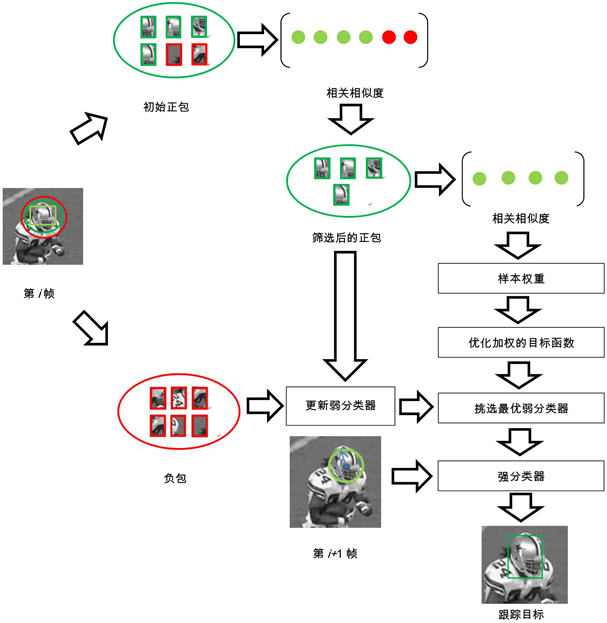Figure 1. SMILE algorithm flow chart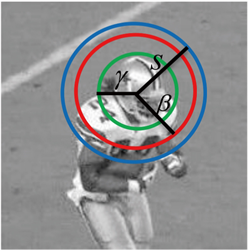Figure 2. Sample range selection

3.2. 相关相似度

$S\left({x}_{ij},{x}_{ik}\right)=0.5\left(NCC\left({x}_{ij},{x}_{ik}\right)+1\right)$ (1)

① 正样本最近邻相似度，也叫正最近邻相似度，

${S}^{+}\left({x}_{ij},E\right)={\mathrm{max}}_{x{}_{1j}\in E}S\left({x}_{ij},{x}_{1j}\right)$ (2)

② 负样本最近邻相似度，也叫负最近邻相似度，

${S}^{-}\left({x}_{11},E\right)={\mathrm{max}}_{{x}_{0j}\in E}S\left({x}_{11},{x}_{0j}\right)$ (3)

③ 相关相似度定义如下：

${S}^{r}\left({x}_{11},E\right)=\frac{{S}^{+}}{{S}^{+}+{S}^{-}}$ (4)

$p\left(y=1|{X}_{1}\right)=\underset{j=1}{\overset{{N}_{1}}{\sum }}{w}_{1j}p\left({y}_{1}=1|{x}_{1j}\right)$ (5)

$p\left(y=0|{X}_{0}\right)=\underset{j=1}{\overset{{N}_{0}}{\sum }}w\left(1-p\left({y}_{0}=1|{x}_{0j}\right)\right)$ (6)

3.3. 本文算法

SMILE算法的具体算法流程如下：

1：选取一组图像块 ${X}^{\text{s}}=\left\{x:‖d\left(x\right)-{d}_{t}^{*}‖ 并计算特征向量。

2：使用SMILE分类器来对 $x\in {X}^{s}$ 估计 $p\left(y|x\right)$

3：更新当前帧的目标位置 ${d}_{t}^{*}=d\left(\mathrm{arg}\mathrm{max}\text{}p\left(y|x\right)\right)$

4：选取两组正包 ${X}_{1}=\left\{x:‖d\left(x\right)-{d}_{t}^{*}‖<\gamma \right\}$ 和负包 ${X}_{0}=\left\{x:\gamma <‖d\left(x\right)-{d}_{t}^{*}‖<\beta \right\}$

5：将包 ${X}_{i}$ 中每一个示例进行归一化，任意两个图像块 ${x}_{ij}$${x}_{ik}$ 的相似度被计算为： $S\left({x}_{ij},{x}_{ik}\right)=0.5\left(NCC\left({x}_{ij},{x}_{ik}\right)+1\right)$

6：计算正包中每个示例的正样本最近邻相似度， ${S}^{+}\left({x}_{1j},E\right)={\mathrm{max}}_{{x}_{1k}\in E}S\left({x}_{1j},{x}_{1k}\right)$，以及负样本最近邻相似度， ${S}^{-}\left({x}_{1j},E\right)={\mathrm{max}}_{{x}_{0k}\in E}S\left({x}_{1j},{x}_{0k}\right)$

7：计算正包中每个示例的相关相似度 ${S}^{r}\left({x}_{1j},E\right)=\frac{{S}^{+}}{{S}^{+}+{S}^{-}}$

8：如果 ${S}^{r}\left({x}_{1j},E\right)\ge \theta$，则图像块 ${x}_{1j}$ 被筛选进新的正包，并计算它的特征向量。

9：利用筛选后的正包、负包中的示例更新弱分类器。

10：计算正包中每个样本的权重 ${w}_{1j}={S}^{r}\left({x}_{1j},E\right)$

11：计算示例概率 $P\left({y}_{i}|{x}_{ij}\right)=\sigma \left(H\left({x}_{ij}\right)\right)=\frac{1}{1+{e}^{-H\left({x}_{ij}\right)}}$

12：根据公式(5)和公式(6)计算加权的包概率。

13：得到 $L=\underset{i}{\sum }\left(\mathrm{log}p\left({y}_{i}|{X}_{i}\right)\right)$ (包的对数似然函数)，通过对它求最大值得到最优的弱分类器， ${h}_{{}_{k}}^{*}=\mathrm{arg}\mathrm{max}L\left({H}_{k-1}+h\right)$

14：重复步骤11~13，直到选出的K个最优的弱分类器依次加入当前强分类器，即 ${H}_{k}\left({x}_{ij}\right)={H}_{k-1}\left({x}_{ij}\right)+{h}_{{}_{k}}^{\text{*}}$

4. 实验Table 1. The information about the seven video sequences

4.1. 参数设置

4.2. 定量分析

4.2.1. 中心位置误差

$\text{CLE}\left(i\right)=\frac{1}{T}\underset{t=1}{\overset{T}{\sum }}\text{dist}\left({\text{center}}_{i}\left(i\right),\text{gt}\left(i\right)\right)$ (7)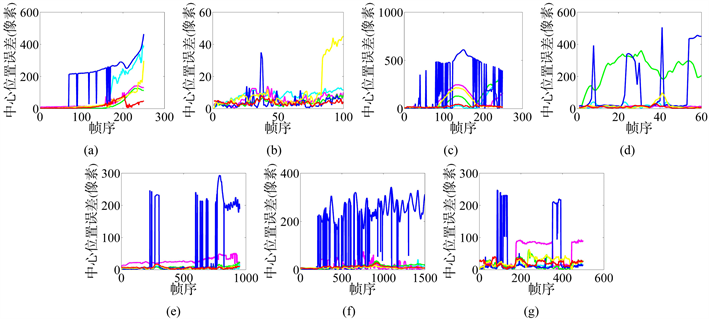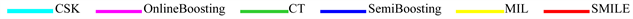Figure 3. Center location error plots for the six trackers and our algorithm. (a) CarScale dataset; (b) Crossing dataset; (c) David3 dataset; (d) Deer dataset; (e) Dog1 dataset; (f) Doll dataset; (g) FaceOcc1 datasetTable 2. Mean center location errors (in pixels) for the six trackers and our algorithm. Bold fonts indicate the best performance while italic fonts indicate the second best

4.2.2. 跟踪精度

$\text{precision}\left(\tau \right)=\frac{1}{T}\underset{t=1}{\overset{T}{\sum }}\left[\frac{1}{R}\underset{r=1}{\overset{R}{\sum }}\delta \left(\text{dist}\left({\text{center}}_{r}\left(i\right),\text{gt}\left(i\right)\right)\le \tau \right)\right]$ (8)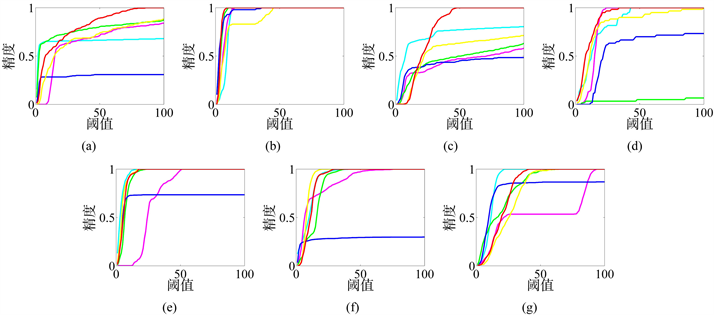Figure 4. Precision plots for the six trackers and our algorithm. (a) CarScale dataset; (b) Crossing dataset; (c) David3 dataset; (d) Deer dataset; (e) Dog1 dataset; (f) Doll dataset; (g) FaceOcc1 dataset

4.3. 定性分析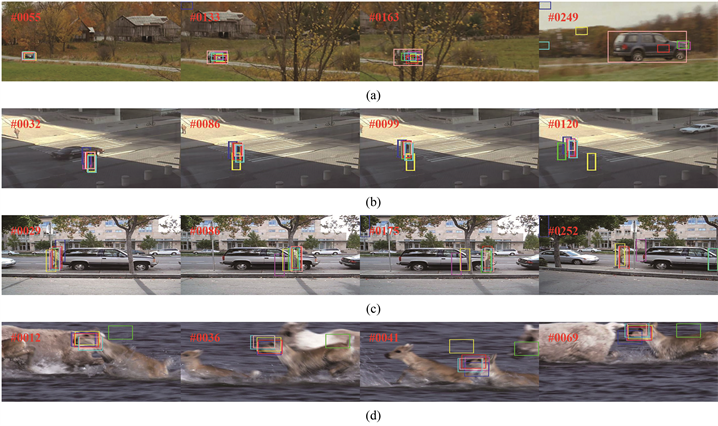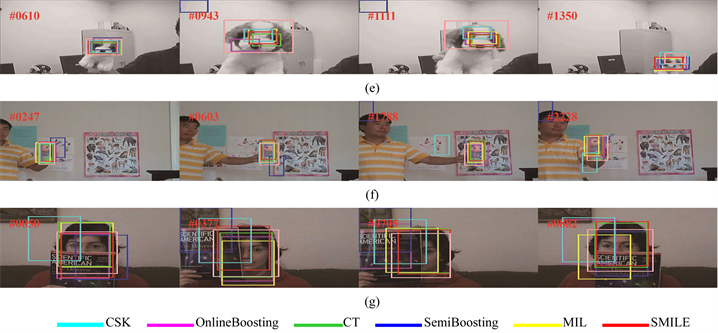Figure 5. Sample tracking results on seven challenging sequences obtained by CSK, Online Boosting, CT, Semi Boosting, MIL and our proposed SMILE algorithms. (a) CarScale dataset; (b) Crossing dataset; (c) David3 dataset; (d) Deer dataset; (e) Dog1 dataset; (f) Doll dataset; (g) FaceOcc1 dataset

5. 结论

  Babenko, B., Yang, M.H. and Belongie, S. (2011) Robust Object Tracking with Online Multiple Instance Learning. IEEE Transactions on Pattern Analysis and Machine Intelligence, 33, 1619-1632. https://doi.org/10.1109/TPAMI.2010.226  Black, M.J. and Jepson, A.D. (1998) Eigen Tracking: Robust Matching and Tracking of Articulated Objects Using a View-Based Representation. IJCV, 26, 63-84.  Avidan, S. (2004) Support Vector Track-ing. IEEE Transactions on Pattern Analysis and Machine Intelligence, 26, 1064-1072. https://doi.org/10.1109/TPAMI.2004.53  Zhang, K. and Song, H. (2013) Real-Time Visual Tracking via Online Weighted Multiple Instance Learning. Pattern Recognition, 46, 397-411. https://doi.org/10.1016/j.patcog.2012.07.013  Li, H.X., Shen, C.N. and Shi, Q. (2011) Real-Time Visual Tracking Using Compressive Sensing. Computer Vision and Pattern Recognition (CVPR), Providence, RI, 20-25 June 2011, 1305-1312.  Havangi, R. (2017) Target Tracking Based on Improved Unscented Particle Filter with Markov Chain Monte Carlo. IETE Journal of Research, 64, 873-885.  Bertinetto, L., Valmadre, J., Golodetz, S., et al. (2016) Staple: Complementary Learners for Real-Time Tracking. Computer Vision & Pattern Recognition, Las Vegas, 26 June-1 July 2016, 1401-1409.  Grabner, H., Leistner, C. and Bischof, H. (2008) Semi-Supervised On-Line Boosting for Robust Tracking. Computer Vision, Marseille, 12-18 October 2008, 234-237. https://doi.org/10.1007/978-3-540-88682-2_19  刘雨情, 肖嵩, 李磊. 在线判别式超像素跟踪算法[J]. 西安电子科技大学学报, 2018, 45(3): 13-17.  Ross, D.A., Lim, J., Lin, R.S., et al. (2008) In-cremental Learning for Robust Visual Tracking. International Journal of Computer Vision, 77, 125-141. https://doi.org/10.1007/s11263-007-0075-7  Mei, X. and Ling, H. (2011) Robust Visual Tracking and Vehicle Classification via Sparse Representation. IEEE Transacti-ons on Pattern Analysis & Machine Intelligence, 33, 2259-2272. https://doi.org/10.1109/TPAMI.2011.66  Vojir, T., Noskova, J. and Matas, J. (2014) Robust Scale-Adaptive Mean-Shift for Tracking. Pattern Recognition Letters, 49, 250-258. https://doi.org/10.1016/j.patrec.2014.03.025  Lytu, N., Letien, T. and Mai, L. (2017) A Study on Particle Filter Based on KLD-Resampling for Wireless Patient Tracking. Industrial Engineering & Management Systems, 16, 92-102. https://doi.org/10.7232/iems.2017.16.1.092  Collins, R.T., Liu, Y. and Leordeanu, M. (2005) Online Selection of Discriminative Tracking Features. IEEE Transactions on Pattern Analysis & Machine Intelligence, 27, 1631-1643. https://doi.org/10.1109/TPAMI.2005.205  Mueller, M., Smith, N. and Ghanem, B. (2017) Context-Aware Correlation Filter Tracking. IEEE Conference on Computer Vision & Pattern Recognition, 1387-1395.  Grabner, H., Grabner, M. and Bischof, H. (2006) Real-Time Tracking via Online Boosting. Proceedings of the British Machine Vision Conference, BMVA, Edinburgh, 47-56.  Bhat, G., Johnander, J., Danelljan, M., et al. (2018) Unveiling the Power of Deep Tracking. European Conference on Computer Vision, Springer, Cham, 493-509.  Cui, Z., Xiao, S., Feng, J., et al. (2016) Recurrently Target-Attending Tracking. 2016 IEEE Conference on Computer Vision and Pattern Recognition (CVPR), IEEE Computer Society, 1449-1458.  李义翠, 亓琳, 谭舒昆. 结合PN约束在线半监督boosting目标跟踪算法[J]. 计算机工程与应用, 2017, 53(23): 129-134.  Nam, H., Baek, M. and Han, B. (2016) Modeling and Propagating CNNs in a Tree Structure for Visual Tracking. European Conference on Computer Vision, Amsterdam, 8-16 October 2016, 1-10.  Viola, P., Platt, J.C. and Zhang, C. (2005) Multiple Instance Boosting for Object Detection. In: International Conference on Neural Information Processing Systems, MIT Press, Cambridge, 1417-1424.  Liu, X. and Yu, T. (2015) Gradient Feature Selection for Online Boosting. 11th International Conference on Com-puter Vision, Rio de Janeiro, 14-21 October 2007, 1-8. https://doi.org/10.1007/978-3-662-48570-5_1  Kalal, Z., Mikolajczyk, K. and Matas, J. (2012) Tracking-Learning-Detection. IEEE Transactions on Pattern Analysis & Machine Intelligence, 34, 1409-1422. https://doi.org/10.1109/TPAMI.2011.239  Wu, Y., Lim, J. and Yang, M.H. (2015) Object Tracking Benchmark. IEEE Transactions on Pattern Analysis and Machine Intelligence, 37, 1834-1848. https://doi.org/10.1109/TPAMI.2014.2388226  Zhang, K., Zhang, L. and Yang, M.H. (2012) Real-Time Compressive Tracking. In: European Conference on Computer Vision, Springer-Verlag, Berlin, 864-877.  Henriques, J.F., Caseiro, R., et al. (2012) Exploiting the Circulant Structure of Tracking-by-Detection with Kernels. In: Computer Vision, Springer, Berlin, Heidelberg, 702-715.  Grabner, H. and Bischof, H. (2006) On-Line Boosting and Vision. IEEE Computer Society Conference on Computer Vision & Pattern Recognition, New York, 17-22 June 2006, Vol. 1, 260-267.  Wu, Y., Lim, J. and Yang, M.H. (2013) Online Object Tracking: A Benchmark. IEEE Conference on Computer Vision and Pattern Recognition, Portland, 23-28 June 2013, Vol. 9, 2411-2418.